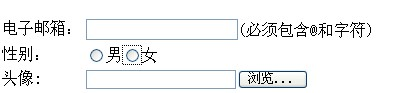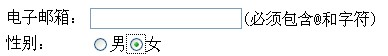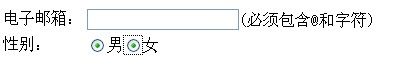# Html: 表单、文本域、单选按钮

form表单判断是那个按钮的提交

<script>
function checkaction(v){
if(v==0){
}else{
}
dbform.submit();
}
</script>
<form action="" method="post" name="formName">
<input type="submit" class="btn" value="提交按钮1" name="opsubmit" οnclick="checkaction(1);" />
<input type="submit" class="btn" value="提交按钮2" name="resubmit" οnclick="checkaction(0);" />
</form>

if((Integer.valueof(request.getparameter("x")))==0){
//按钮2被按下时提交内容的处理程序
}else｛
//按钮1被按下时提交内容的处理程序

<textarea id="mytext" value="请在此输入文本内容" οnfοcus="if (value =='请在此输入文本内容'){value =''}"></textarea>

1.必须这该组的Raido 的name属性赋值，该组中的单选按钮才能被选中，例如：表单中截取如下代码：name属性为空；

<tr>

<td>性别：</td>

</tr>2.修改代码：给name属性都赋值“gender”

<tr>
<td>性别：</td>
</tr>3.修改代码：给Name属性分别赋不同的值“gender” 和“sex”：

<tr>

<td>性别：</td>
</tr>JS命名规则

var aPerson = [];// Array数组

var oBtn = document.getElementById('btn');//Object对象

var fnName =function() {};// function函数

var sName ="w3cplus";// string字符串

©️2019 CSDN 皮肤主题: 编程工作室 设计师: CSDN官方博客